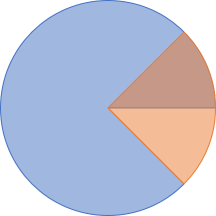In geometry, an angle is a measure of how two crossing lines relate to each other. In mathematics, angles are usually represented in radians, but in daily use, they're mostly measured in degrees between 0 and 360.

You can always draw an angle within a circle. Here's a 45° angle:If you add another 90° angle to that, you get a 135° angle:What do you get if you add 90° to 315°?Well, you get 45°, of course!There's only 360° in a circle, so overflow is handled, in this case, by subtracting 360°. In general, however, angular addition is nothing but modulo 360 addition.

### Angle struct #

You can model a geometric angle as a struct. Here's a simple example:

```public struct Angle
{

private Angle(decimal degrees)
{
this.degrees = degrees % 360m;
if (this.degrees < 0)
this.degrees += 360m;
}

public static Angle FromDegrees(decimal degrees)
{
return new Angle(degrees);
}

{
return new Angle((decimal)((180D / Math.PI) * radians));
}

{
return new Angle(this.degrees + other.degrees);
}

public readonly static Angle Identity = new Angle(0);

public override bool Equals(object obj)
{
if (obj is Angle)
return ((Angle)obj).degrees == this.degrees;

return base.Equals(obj);
}

public override int GetHashCode()
{
return this.degrees.GetHashCode();
}

public static bool operator ==(Angle x, Angle y)
{
return x.Equals(y);
}

public static bool operator !=(Angle x, Angle y)
{
return !x.Equals(y);
}
}```

Notice the `Add` method, which is a binary operation; it's an instance method on `Angle`, takes another `Angle` as input, and returns an `Angle` value.

### Associativity #

Not only is `Add` a binary operation; it's also associative. Here's an example:

```var x = Angle.FromDegrees(135);
var y = Angle.FromDegrees(180);
var z = Angle.FromDegrees(300);

Notice that `left` first evaluates `x.Add(y)`, which is 315°; then it adds 300°, which is 615°, but normalises to 255°. On the other hand, `right` first evaluates `y.Add(z)`, which is 480°, but normalises to 120°. It then adds those 120° to `x`, for a final result of 255°. Since `left` and `right` are both 255°, this illustrates that `Add` is associative.

Obviously, this is only a single example, so it's no proof. While still not a proof, you can demonstrate the associativity property with more confidence by writing a property-based test. Here's one using FsCheck and xUnit.net:

```[Property(QuietOnSuccess = true)]
public void AddIsAssociative(Angle x, Angle y, Angle z)
{
Assert.Equal(
}```

By default, FsCheck generates 100 test cases, but even when I experimentally change the configuration to run 100,000 test cases, they all pass. For full disclosure, however, I'll admit that I defined the data generators to only use `NormalFloat` for the radian values, and only `decimal` values with up to 10 decimal places. If you try to use entirely unconstrained floating points, you'll see test failures caused by rounding errors.

Changing the data generator is one way to address rounding errors. Another way is to add a bit of fuzzy tolerance to the assertion. In any case, though, the `Add` operation is associative. That rounding errors occur is an implementation detail of floating point arithmetic.

### Identity #

The above code listing defines a value called `Identity`:

`public readonly static Angle Identity = new Angle(0);`

As an Angle, I want my Add and Identity members to obey the monoid laws so that I can be a monoid.

As an example, both `left` and `right` should be `true` in the following:

```var x = Angle.FromDegrees(370);

var left  = x == Angle.Identity.Add(x);
var right = x == x.Add(Angle.Identity);```

That does, indeed, turn out to be the case.

Again, you can generalise using FsCheck:

```[Property(QuietOnSuccess = true)]
{
}```

Once more, a reservation identical to the one given above must be given when it comes to floating point arithmetic.

### Conclusion #

The `Add` method is an associative, binary operation with identity; it's a monoid.

As far as I can tell, any modulo-based addition is a monoid, but while, say, modulo 37 addition probably doesn't have any practical application, modulo 360 addition does, because it's how you do angular addition.

### Wish to comment?

You can add a comment to this post by sending me a pull request. Alternatively, you can discuss this post on Twitter or somewhere else with a permalink. Ping me with the link, and I may respond.

#### Published

Monday, 16 July 2018 14:40:00 UTC

#### Tags

"Our team wholeheartedly endorses Mark. His expert service provides tremendous value."
Hire me!
Published: Monday, 16 July 2018 14:40:00 UTC